# The Hexagonal Antiprism

The hexagonal antiprism is a 3D uniform polyhedron bounded by 14 polygons (2 hexagons and 12 triangles), 24 edges, and 12 vertices.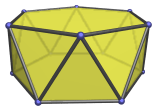The height of a hexagonal antiprism with an edge length of 2 is 2√(√3−1), or approximately 1.711199.

The hexagonal antiprism can be attached to a triangular cupola to form a gyroelongated triangular cupola (J22), a Johnson solid. Adding another cupola on the other side of the antiprism produces the gyroelongated triangular bicupola (J44).

## Projections

Here are some views of the hexagonal antiprism from various angles:

Projection Envelope Description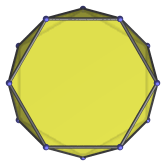Regular dodecagon

Parallel projection centered on hexagonal face.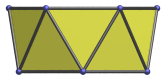Trapezium

Parallel projection parallel to square faces and a pair of triangles.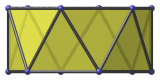Rectangle

Parallel projection centered on an edge between two triangles.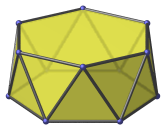Heptagon

Vertex-centered parallel projection.

## Coordinates

The Cartesian coordinates of the hexagonal antiprism, centered on the origin and having edge length 2, are:

• (±1, ±√3, √(√3−1))
• (±2, 0, √(√3−1))
• (±√3, ±1, −√(√3−1))
• (0, ±2, −√(√3−1))

Last updated 17 Jun 2019.